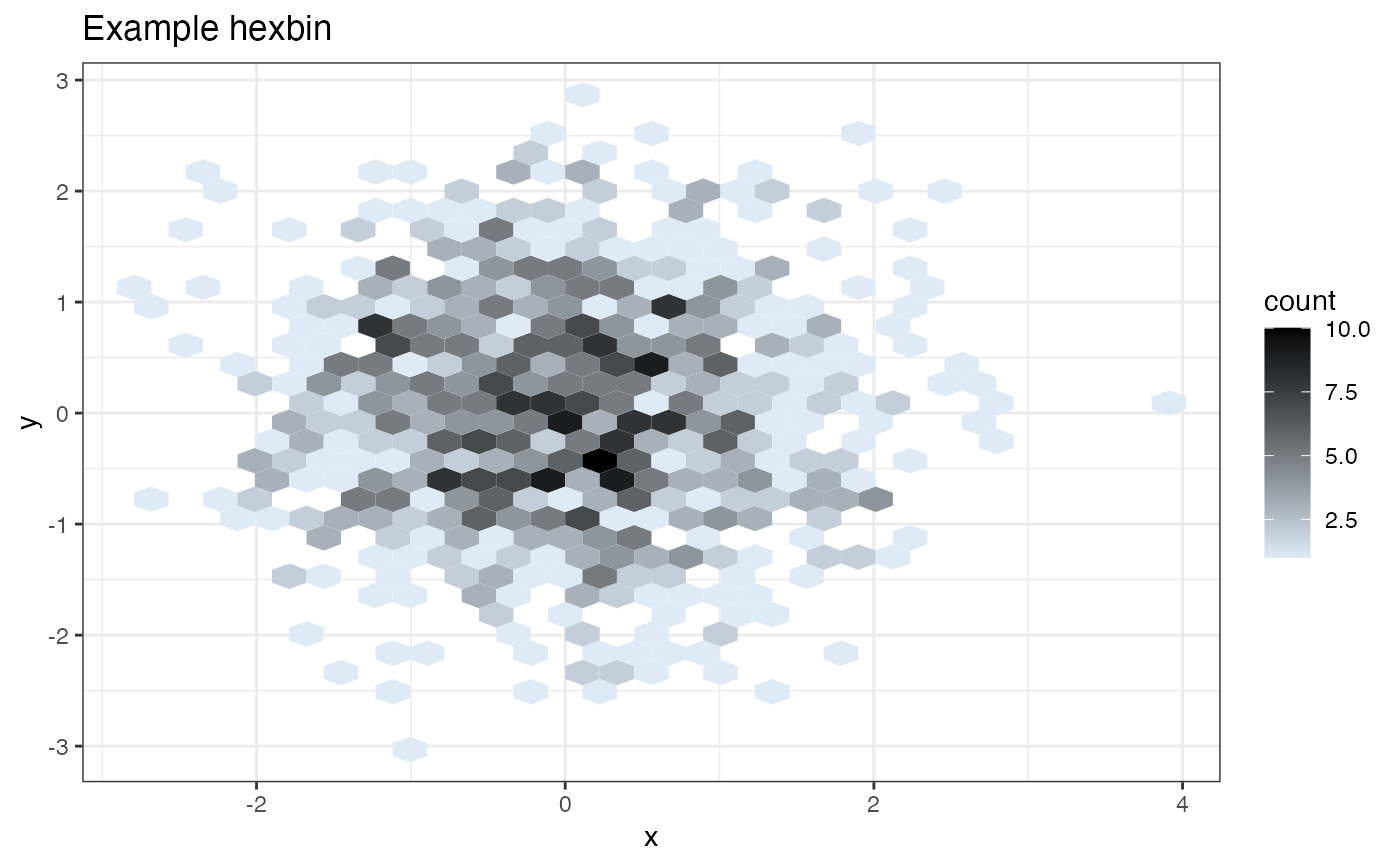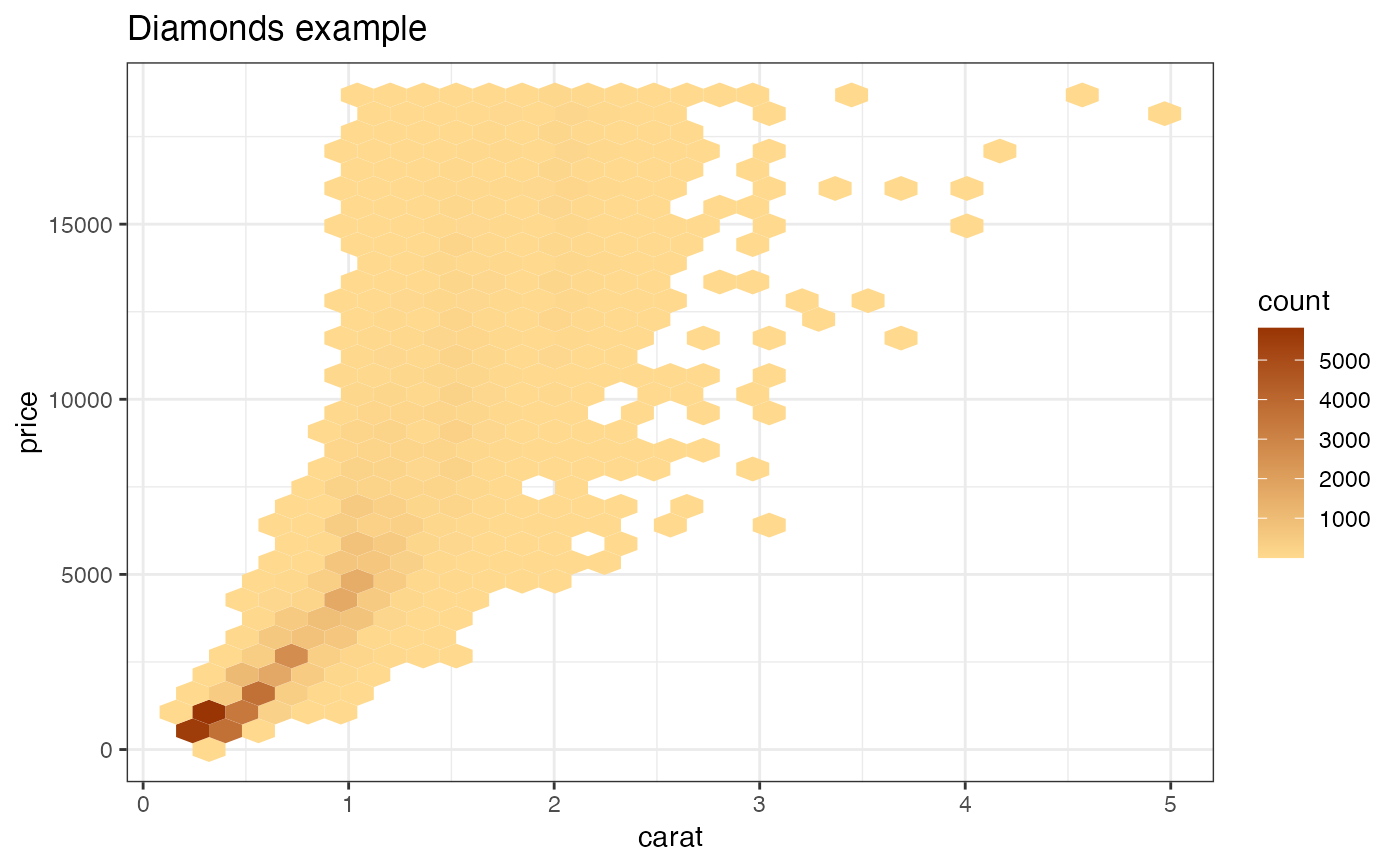Build a hex bin plot with rational color coding.

HexBinPlot(
d,
xvar,
yvar,
title,
...,
lightcolor = "#deebf7",
darkcolor = "#000000",
bins = 30,
binwidth = NULL,
na.rm = FALSE
)

## Arguments

d data frame name of x variable column name of y variable column plot title not used, forces later arguments to bind by name light color for least dense areas dark color for most dense areas passed to geom_hex passed to geom_hex passed to geom_hex

## Value

a ggplot2 hexbin plot

## Details

Builds a standard ggplot2 hexbin plot, with a color scale such that dense areas are colored darker (the default ggplot2 fill scales will color dense areas lighter).

The user can choose an alternate color scale with endpoints lightcolor and darkcolor; it is up to the user to make sure that lightcolor is lighter than darkcolor.

Requires the hexbin package.

geom_hex

## Examples


if(requireNamespace("hexbin", quietly = TRUE)) {
set.seed(634267)
dframe = data.frame(x = rnorm(1000), y = rnorm(1000))
print(HexBinPlot(dframe, "x", "y", "Example hexbin"))

diamonds = ggplot2::diamonds
print(HexBinPlot(diamonds, "carat", "price", "Diamonds example"))

# change the colorscale
print(HexBinPlot(diamonds, "carat", "price", "Diamonds example",
lightcolor="#fed98e",
darkcolor="#993404"))
}